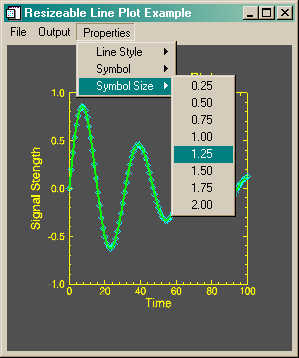Sizing Symbols in IDL Object Graphics

QUESTION: I can't seem to get my symbols sized appropriately in my object graphics plot. They often appear in distorted shapes. Can you show me a method to size symbols in a way that preserves their shape?ANSWER: Here is a method for setting the symbol size that seems to work reasonably well under all the conditions in which I have tested it.

The key is to set the symbol size based upon the same relative proportion of the axis length, in data coordinate space. For example, I find that 0.015 times the data range is a nice symbol size. So, if the axis goes from 0 to 100, the symbol size would be 1.5. If the axis goes from 0 to 1, the symbol size would be 0.015.

In practice, I get the axis ranges from the axis objects after I have created them, and use these values to calculate a symbol size. The code looks something like this:

xAxis->GetProperty, CRange=xrange
yAxis->GetProperty, CRange=yrange

; Size the symbols appropriately for the plot.

xSymSize = (xrange - xrange) * 0.015
ySymSize = (yrange - yrange) * 0.015
thisSymbol->SetProperty, Size=[xSymSize, ySymSize]

You can find an example of how symbols are sized and used in the XPLOT program from the Coyote Library. The illustration below shows the program running with triangle symbols at the default symbol size.

IDL> XPlot, Findgen(11), PSym=5Web Coyote's Guide to IDL Programming